### Home > MC2 > Chapter 10 > Lesson 10.1.2 > Problem10-18

10-18.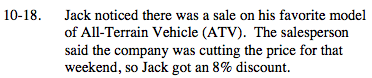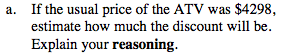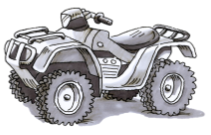Round the ATV price to $4300 in order to make the percent easier to approximate. Since we can find that 10% of$4300 is $430, we can also deduce that 5% of$4300 would be $215. 8% is roughly in the middle of 10% and 5%. What is your estimation?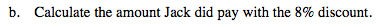Find 8% of$4298, then subtract that value from $4298 to find how much Jack paid. 4298 − 343.84 = 4298(.08) = 343.84$3954.16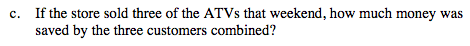For each ATV, the original price was $4298. Based on part (b), it costs$3954.16 after the 8% discount. How much did Jack save on one ATV? Now multiply that number by 3.